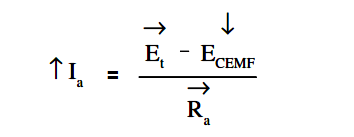# DC Motor Speed

The field of a DC motor is varied using external devices, usually field resistors. For a constant applied voltage to the field (E), as the resistance of the field (Rf) is lowered, the amount of current flow through the field (If) increases as shown by Ohm’s law in below Equation.An increase in field current will cause field flux (Φf ) to increase. Conversely, if the resistance of the field is increased, field flux will decrease. If the field flux of a DC motor is decreased, the motor speed will increase. The reduction of field strength reduces the CEMF of the motor, since fewer lines of flux are being cut by the armature conductors, as shown in below Equation.A reduction of counter EMF allows an increase in armature current as shown in below Equation.This increase in armature current causes a larger torque to be developed; the increase in armature current more than offsets the decrease in field flux as shown in below Equation.This increased torque causes the motor to increase in speed.This increase in speed will then proportionately increase the CEMF. The speed and CEMF will continue to increase until the armature current and torque are reduced to values just large enough to supply the load at a new constant speed.